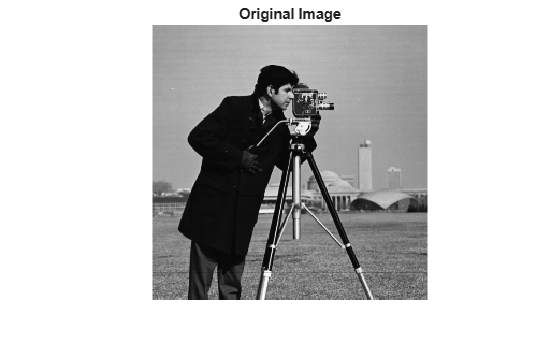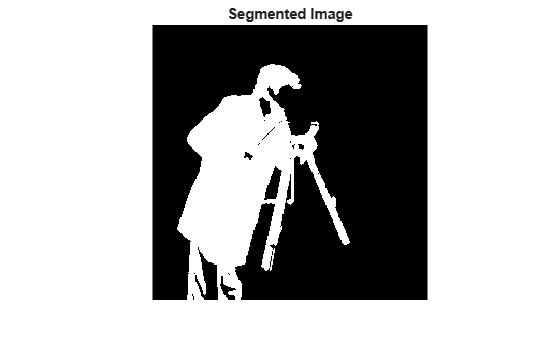# graydiffweight

Calculate weights for image pixels based on grayscale intensity difference

## Syntax

``W = graydiffweight(I,refGrayVal)``
``W = graydiffweight(I,mask)``
``W = graydiffweight(I,C,R)``
``W = graydiffweight(V,C,R,P)``
``W = graydiffweight(___, Name,Value)``

## Description

````W = graydiffweight(I,refGrayVal)` computes the pixel weight for each pixel in the grayscale image `I`. The weight is the absolute value of the difference between the intensity of the pixel and the reference grayscale intensity specified by the scalar `refGrayVal`. Pick a reference grayscale intensity value that is representative of the object you want to segment. The weights are returned in the array `W`, which is the same size as input image `I`. The weight of a pixel is inversely related to the absolute value of the grayscale intensity difference at the pixel location. If the difference is small (intensity value close to `refGrayVal`), the weight value is large. If the difference is large (intensity value very different from `refGrayVal`), the weight value is small.```
````W = graydiffweight(I,mask)` computes the pixel weights, where the reference grayscale intensity value is the average of the intensity values of all the pixels in `I` that are marked as logical `true` in `mask`. Using the average of several pixels to calculate the reference grayscale intensity value can be more effective than using a single reference intensity value, as in the previous syntax.```
````W = graydiffweight(I,C,R)` computes the pixel weights, where the reference grayscale intensity value is the average of the intensity values of the pixel locations specified by the vectors `C` and `R`. `C` and `R` contain the column and row indices of the pixel locations that must be valid pixel indices in `I`.```
````W = graydiffweight(V,C,R,P)` computes the weights for each voxel in the volume `V`, specified by the vectors `C`, `R`, and `P`. `C`, `R`, and `P` contain the column, row, and plane indices of the voxel locations that must be valid voxel indices in `V`.```

example

````W = graydiffweight(___, Name,Value)` returns the weight array `W` using name-value pairs to control aspects of weight computation.```

## Examples

collapse all

This example segments an object in an image using Fast Marching Method using grayscale intensity difference weights calculated from the intensity values at the seed locations.

Read image and display it.

```I = imread('cameraman.tif'); imshow(I) title('Original Image')```Specify row and column index of pixels for use a reference grayscale intensity value.

```seedpointR = 159; seedpointC = 67;```

Calculate the grayscale intensity difference weight array for the image and display it. The example does log-scaling of `W` for better visualization.

```W = graydiffweight(I, seedpointC, seedpointR,'GrayDifferenceCutoff',25); figure, imshow(log(W),[])```Segment the image using the grayscale intensity difference weight array. Specify the same seed point vectors you used to create the weight array.

```thresh = 0.01; BW = imsegfmm(W, seedpointC, seedpointR, thresh); figure, imshow(BW) title('Segmented Image')```## Input Arguments

collapse all

Grayscale image, specified as a 2-D numeric matrix.

Data Types: `single` | `double` | `int8` | `uint8` | `int16` | `uint16` | `int32` | `uint32`

Grayscale volume, specified as a 3-D numeric array.

Data Types: `single` | `double` | `int8` | `uint8` | `int16` | `uint16` | `int32` | `uint32`

Reference grayscale intensity value, specified as a scalar.

Data Types: `double`

Reference grayscale intensity mask, specified as a logical array of the same size as `I`.

Data Types: `logical`

Column index of reference pixel (or voxel), specified as a numeric (integer-valued) vector.

Data Types: `double`

Row index of reference pixel (or voxel), specified as a numeric (integer-valued) vector.

Data Types: `double`

Plane index of reference voxel, specified as a numeric (integer-valued) vector.

Data Types: `double`

### Name-Value Arguments

Specify optional pairs of arguments as `Name1=Value1,...,NameN=ValueN`, where `Name` is the argument name and `Value` is the corresponding value. Name-value arguments must appear after other arguments, but the order of the pairs does not matter.

Before R2021a, use commas to separate each name and value, and enclose `Name` in quotes.

Example: `W = graydiffweight(I, seedpointC, seedpointR,'GrayDifferenceCutoff',25);`

Output weight roll-off factor, specified as the comma-separated pair consisting of `'RolloffFactor'` and a positive scalar of class `double`. Controls how fast the output weight falls as the function of the absolute difference between an intensity value and the reference grayscale intensity. When viewed as a 2-D plot, pixel intensity values can vary gradually at the edges of regions, creating a gentle slope. In your segmented image, you might want the edge to be more well-defined. Using the roll-off factor, you control the slope of the weight value curve at points where intensity values start to change. If you specify a high value, the output weight values fall off sharply around the regions of change intensity. If you specify a low value, the output weight has a more gradual fall-off around the regions of changing intensity. The suggested range for this parameter is `[0.5 4]`.

Data Types: `double`

Threshold for absolute grayscale intensity difference values, specified as the comma-separated pair consisting of `'GrayDifferenceCutoff'` and a nonnegative scalar of class `double`. When you put a threshold on intensity difference values, you strongly suppress output weight values greater than the cutoff value. `graydiffweight` assigns these pixels the smallest weight value. When the output weight array `W` is used for Fast Marching Method based segmentation (as input to `imsegfmm`), this parameter can be useful in improving the accuracy of the segmentation output. Default value of this parameter is `Inf`, which means that there is no hard cutoff.

Data Types: `double`

## Output Arguments

collapse all

Weight array, specified as numeric array of the same size as the input image `I` or volume `V`. `W` is of class `double`, unless the input image or volume is of class `single`, in which case `W` is of class `single`.

## Version History

Introduced in R2014b